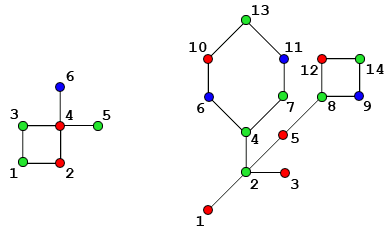시간 제한 메모리 제한 제출 정답 맞은 사람 정답 비율
2 초 256 MB 1 1 1 100.000%

## 문제

Cactus is a connected undirected graph in which every edge belongs to at most one simple cycle.

You are given a cactus having $n$ vertices and $m$ edges. Each vertex of this cactus is either red, green or blue. All the vertices are numbered with sequential positive integers from $1$ to $n$. The examples of such graphs are shown in the figure:Initially, you are standing at the vertex $s$. Then you start to move to some adjacent vertex, which hasn't been visited before, until no such vertex exists. The choice of each eligible vertex is equiprobable. When there is no such vertex, you are stopped at some vertex $t$. If $t$ is colored red or green --- the process is stopped. If $t$ is colored blue --- the process should be restarted from the vertex $s$ again.

You are to write the program that for given cactus computes the probability $\frac{p}{q}$ that the process will be stopped when you are standing at a red vertex and outputs integer $p \cdot q^{-1} \mod 1000000007$ ($10^{9}+7$). Here $q^{-1}$ is a modular multiplicative inverse of an integer $q$ modulo $1000000007$.

## 입력

The first line of input contains three space-separated integers $n$, $m$ and $s$ ($2 \leq n \leq 10^{5}$, $m \geq n - 1$, $1 \leq s \leq n$). The second line of input contains $n$ characters, $i$-th of them denotes the color of vertex $i$: 'R' denotes red, 'G' --- green and 'B' --- blue. Each of the following $m$ lines contains two integers $u_{i}$, $v_{i}$ --- the numbers of vertices connected by the edge ($1 \leq u_{i}, v_{i} \leq n$).

It is guaranteed that the given graph is a cactus and it contains no multiple edges.

## 출력

The only line of output must contain one integer $p \cdot q^{-1} \bmod 10^9+7$. If the process is infinite, output "NaN" instead (quotes for clarity).

## 예제 입력 1

6 6 1
GRGRGB
1 2
1 3
2 4
3 4
4 5
4 6


## 예제 출력 1

250000002


## 예제 입력 2

14 15 2
RGRGRBGGBRBRGG
1 2
2 3
2 4
5 2
7 4
4 6
10 6
11 7
10 13
11 13
8 5
8 12
8 9
14 9
12 14


## 예제 출력 2

833333340


## 힌트

Both samples correspond to the image in the statement. The first sample corresponds to the left cactus and the second sample --- corresponds to the right one.